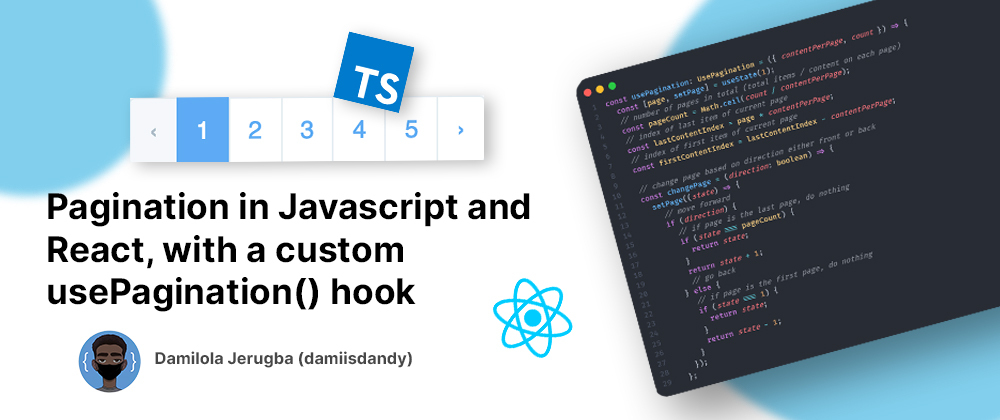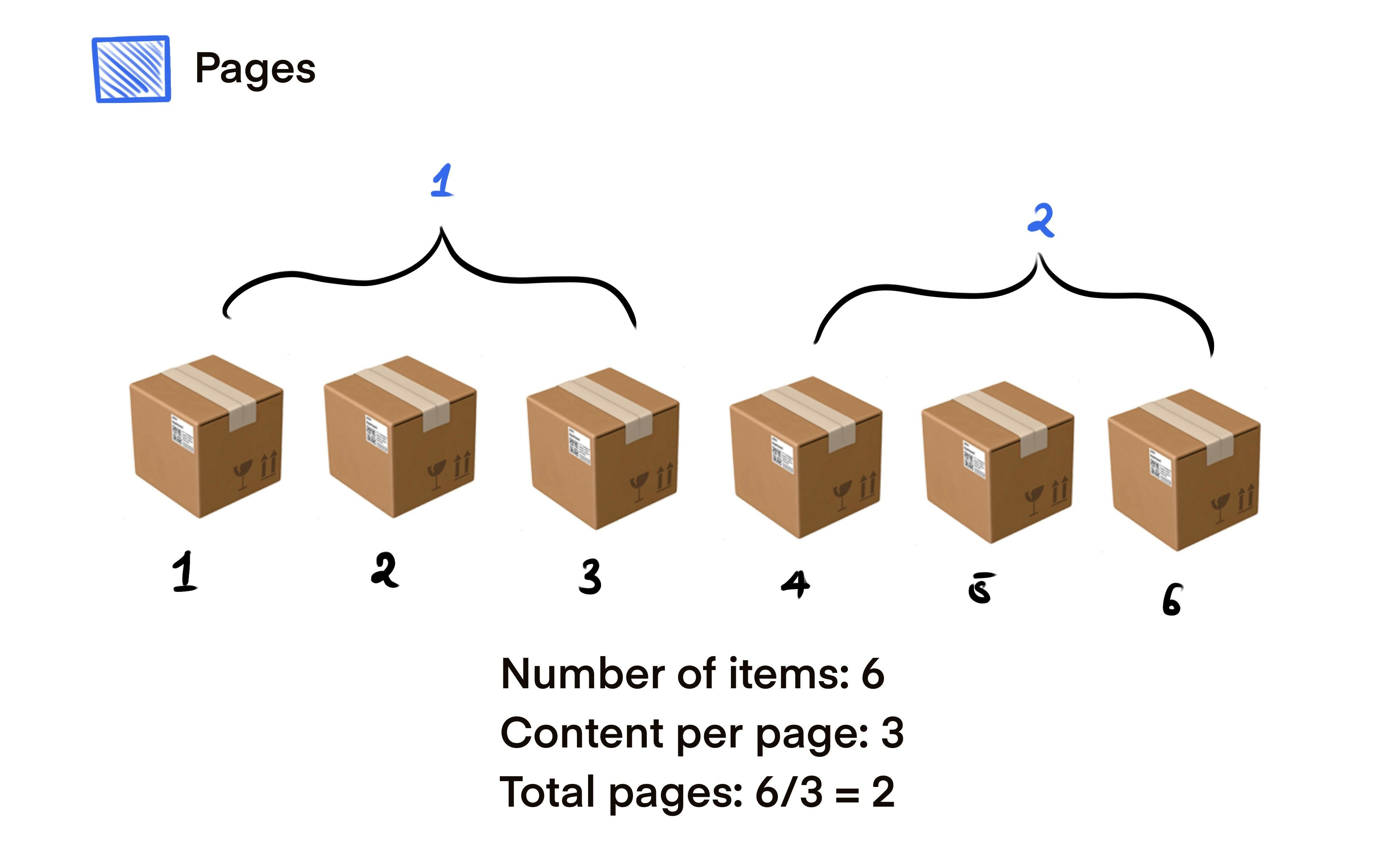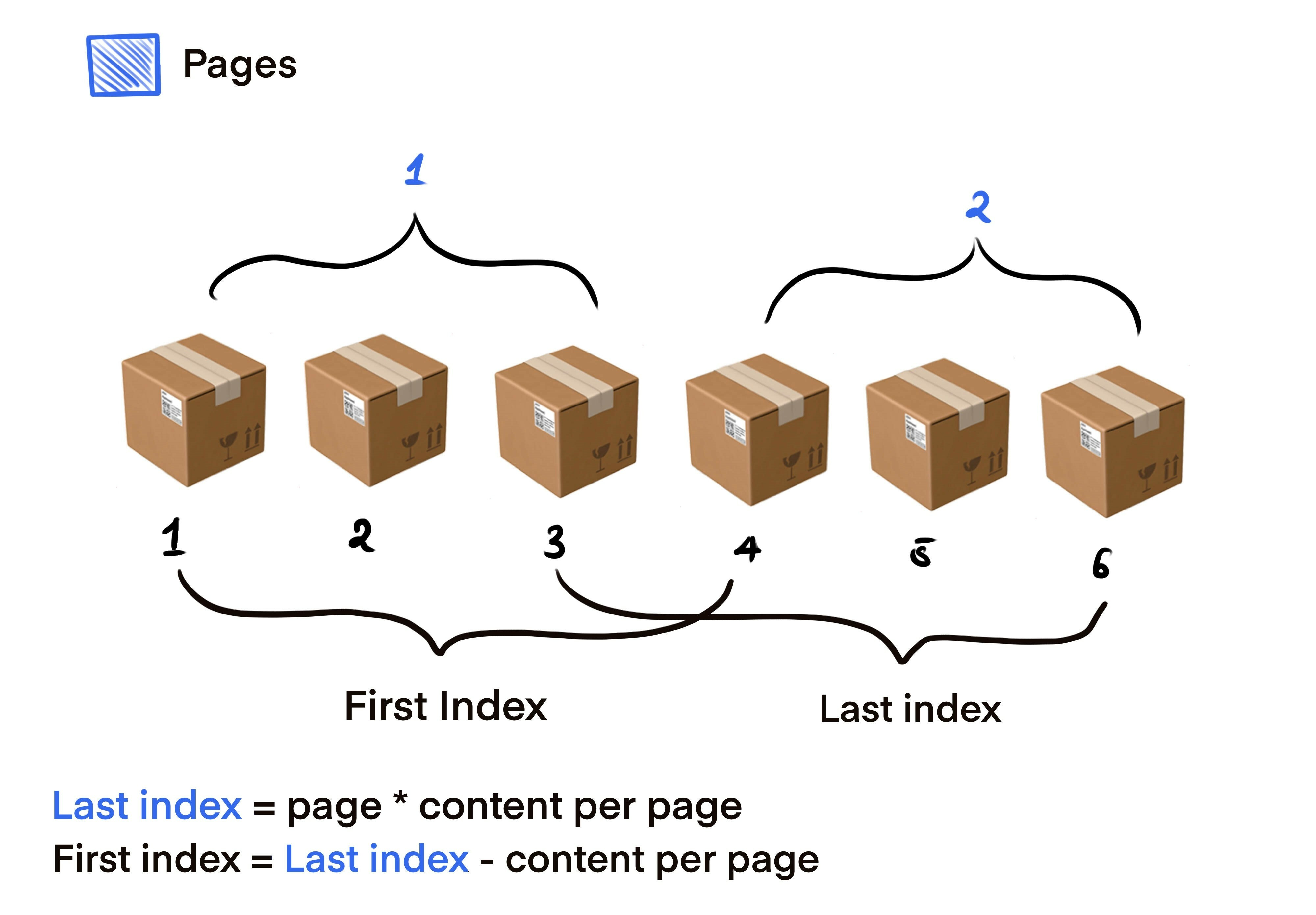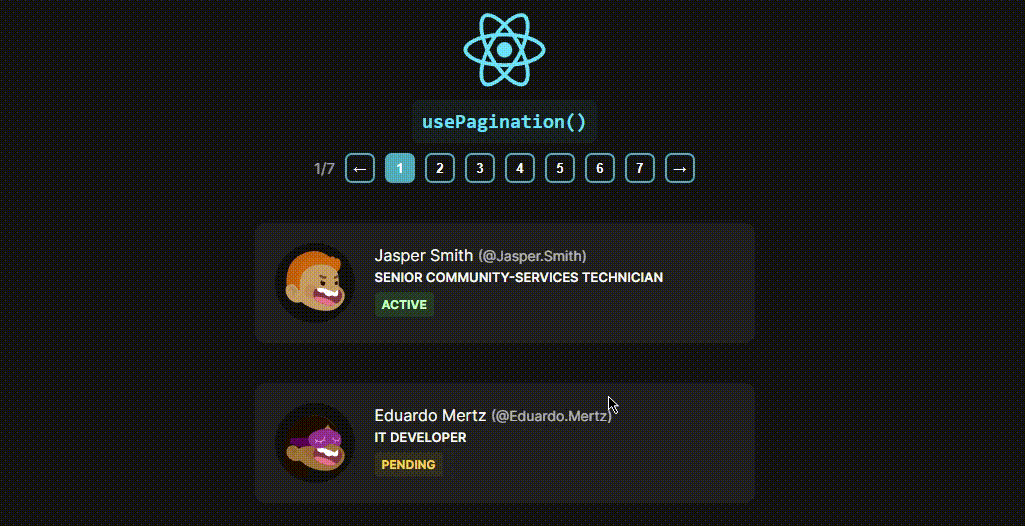# Pagination in Javascript and React, with a custom usePagination() hookThis guide is to help you understand the concept of pagination and how to implement it in react, the concepts in this tutorial can be applied to any javascript project.

## 🤨 What is Pagination?

Pagination is the process of separating print or digital content into discrete pages. For print documents and some online content, pagination also refers to the automated process of adding consecutive numbers to identify the sequential order of pages.## Concept behind it? 💻

Let’s say you have a total of 6 items on a page, and you want to only display 3 items at a time (per page). This means we are going to have a total of 2 pages, and if we want to display 2 items per page this means a total of?? you guessed it! 3 pages.This formular is rather simple: `totalPages = totalContent / contentPerPage`

## Implementing it in Javascript (.slice()) 🔪

Calculating the content per page is rather easy, but how do we display certain content based on what page we are on? We simply need to understand the relationship between the page and the `index` of our content. Let first understand the `.slice()` Array method.

The slice() method returns a shallow copy of a portion of an array into a new array object selected from start to end (end not included) where start and end represent the index of items in that array. The original array will not be modified.

For example, let’s say we have an array called `scouts` and we want to select only a portion of this array based on the array’s index.

``````const scouts = ['levi', 'hange', 'erwin', 'petra', 'oruo', 'miche'];
scouts.slice(2, 5);
// output: [ 'erwin', 'petra', 'oruo' ]
scouts.slice(1, 3);
// output: [ 'hange', 'erwin' ]``````

We all know javascript follows a zero-based index, so the first parameter is the index from which we want to start the slice from and the second parameter is the index right after where we want the slice to end. e.g if we want 2 to 4 we use `.slice(2, 5)` as seen in the first example.

## Mapping page number to index

All we need to do is know what the `startIndex` and `lastIndex` should be based on the page number. this relationship is quite simple.As you can tell from the diagram above the last index is simply the current page multiplied by the given content by page, while the first index is the content by page subtracted from the last index.

``````// assuming we are on page one
const page = 1;
const contentPerPage = 3;
const lastIndex = page * contentPerPage; // 3
const firstIndex = lastIndex - contentPerPage; // 0

scouts.slice(firstIndex, lastIndex);
// scouts.slice(0, 3) => [ 'levi', 'hange', 'erwin' ]

// page 2
// scouts.slice(3, 6) => [ 'petra', 'oruo', 'miche' ]``````

Wow!, that was easy 😳.

## Custom `usePagination` hook 🎣

Now that we’ve learned the concept behind it, let’s implement this in react and create our custom hook to help us automate this process. This hook takes in an object that takes in the properties `contentPerPage` which is how many items should be displayed at a time and `count` which is the total number of items given (Array length). It also returns an object with the following properties.

• `page` - current page we are on
• `totalPages` - total number of pages generated
• `firstContentIndex` - first index for the `.slice()` method
• `lastContentIndex` - last index for the `.slice()` method
• `nextPage` - function to navigate one page foward
• `prevPage` - function to navigate one page backward
• `setPage` - function to go to a certain page

The type definitions are as follows:

``````interface UsePaginationProps {
contentPerPage: number;
count: number;
}

interface UsePaginationReturn {
page: number;
totalPages: number;
firstContentIndex: number;
lastContentIndex: number;
nextPage: () => void;
prevPage: () => void;
setPage: (page: number) => void;
}

type UsePagination = UsePaginationProps => UsePaginationReturn;``````

In your React project create a folder called `hooks` and create a file called `usePagination`, this is where our custom hook will reside.

Type the following within it

``````import { useState } from 'react';

const usePagination: UsePagination = ({ contentPerPage, count }) => {
const [page, setPage] = useState(1);
// number of pages in total (total items / content on each page)
const pageCount = Math.ceil(count / contentPerPage);
// index of last item of current page
const lastContentIndex = page * contentPerPage;
// index of first item of current page
const firstContentIndex = lastContentIndex - contentPerPage;

// change page based on direction either front or back
const changePage = (direction: boolean) => {
setPage(state => {
// move forward
if (direction) {
// if page is the last page, do nothing
if (state === pageCount) {
return state;
}
return state + 1;
// go back
} else {
// if page is the first page, do nothing
if (state === 1) {
return state;
}
return state - 1;
}
});
};

const setPageSAFE = (num: number) => {
// if number is greater than number of pages, set to last page
if (num > pageCount) {
setPage(pageCount);
// if number is less than 1, set page to first page
} else if (num < 1) {
setPage(1);
} else {
setPage(num);
}
};

return {
totalPages: pageCount,
nextPage: () => changePage(true),
prevPage: () => changePage(false),
setPage: setPageSAFE,
firstContentIndex,
lastContentIndex,
page,
};
};

export default usePagination;``````

We are managing the current page value with `useState`, also notice that `pageCount` is also equal to the value of the last page. I’ve made the code above as explanatory as I can.

## Implementation ✍🏾

We simply import the hook then input the needed properties.

``````...
const {
firstContentIndex,
lastContentIndex,
nextPage,
prevPage,
page,
setPage,
totalPages,
} = usePagination({
contentPerPage: 3,
count: people.length,
});
...``````

Then we simply slice our data with the `firstContentIndex` and `lastContentIndex`.

``````...
<div className="items">
{people
.slice(firstContentIndex, lastContentIndex)
.map((el: any) => (
<div className="item" key={el.uid}></div>
))}
</div>
...``````

Below is a simple functionality to help us generate our buttons, then we add their corresponding `onClick` handlers.

``````<div className="pagination">
<p className="text">
{page}/{totalPages}
</p>
<button onClick={prevPage} className="page">
&larr;
</button>
{/* @ts-ignore */}
{[...Array(totalPages).keys()].map(el => (
<button
onClick={() => setPage(el + 1)}
key={el}
className={`page \${page === el + 1 ? 'active' : ''}`}
>
{el + 1}
</button>
))}
<button onClick={nextPage} className="page">
&rarr;
</button>
</div>``````

We are done! As you can see below our `usePagination` hook works as planned.Thank you for reading 🙏🏾, If you have any questions, additions, or subtractions please comment below.

The full source code is linked below 👇👇